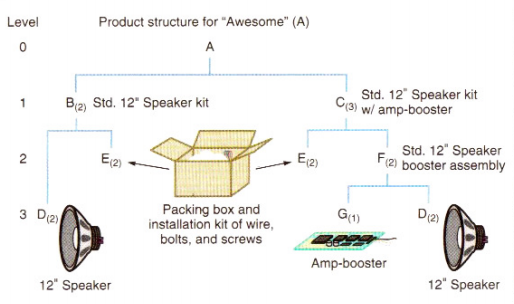# Solved: Can someone explain to me how do we define what week to place a number for the required

Can someone explain to me how do we define what week to place a number for the required dates?

I understand part A but the other parts seem confusing.

Don't use plagiarized sources. Get Your Custom Essay on
Solved: Can someone explain to me how do we define what week to place a number for the required
GET AN ESSAY WRITTEN FOR YOU FROM AS LOW AS \$13/PAGE

For example, why do part B and C both have a required date in week 7?

Why do part E and D both have a required date in week 6?

Gross Requirements Plan Gross Material Requirements Plan for 50 Awesome Speaker Kits (As) with Order Release Dates Also Shown TABLE 14.3 WEEK TIME A. Required date 50 Order release date 50 1 week B. Required date 100 Order release date 100 2 weeks C. Required date 150 Order release date 150 1 week E. Required date 200 Order release date 200 300 2 weeks F. Required date 300 Order release date 300 3 weeks D. Required date 600 200 Order release date 600 200 1 week G. Required date 300 Order release date 300 2 weeks © 2014 Pearson EducationInc 14-34 ,Note that as per the product structure,

two(2) units of B are fitted in one(1) unit of A
& three(3) units of C are fitted in one(1) unit of A.

Since B. and C are just at the lower hierarchy of ‘A’ in the product structure, the week when the order release of A happens, the required date of B and C happens.

So, since the order release of A happens in week 7, the required date of B, and C is in the same week (i.e. week 7). The numbers are as per the units required i.e. No. of B = 2 x No. of A = 2 x 50 = 100 and No. of C=3 x 50 = 150.

——————–

E, and D are more complicated. But the logic is the same as above.

——————–

Consider ‘E’ first.

Two(2) units of E are fitted in one(1) unit of B

Since E is just at the lower hierarchy of B in the product structure, the week when the order release of B happens, the required date of E happens.

So, since the order release of B happens in week 5, the required date of E is in the same week (i.e. week 5). The numbers are as per the units required i.e. No. of E = 2 x No. of B = 2 x 100 = 200

So, for making ‘B’ only, we need 200 units of E in week 5 ————————–1

Two(2) units of E are fitted in one(1) unit of C

Since E is just at the lower hierarchy of C in the product structure, the week when the order release of C happens, the required date of E happens.

So, since the order release of C happens in week 6, the required date of E is in the same week (i.e. week 6). The numbers are as per the units required i.e. No. of E = 2 x No. of C = 2 x 150 = 300

So, for making ‘C’ only, we need 300 units of E in week 6 ————————– 2

Combine 1 and 2 to get the overall requirement of E i.e. 200 in week 5 and 300 in week 6

——————————–

Consider ‘D’ now

Two(2) units of D are fitted in one(1) unit of B

Since D is just at the lower hierarchy of B in the product structure, the week when the order release of B happens, the required date of D happens.

So, since the order release of B happens in week 5, the required date of D is in the same week (i.e. week 5). The numbers are as per the units required i.e. No. of D = 2 x No. of B = 2 x 100 = 200

So, for making ‘B’ only, we need 200 units of D in week 5 ————————–3

Two(2) units of D are fitted in one(1) unit of F

Since D is just at the lower hierarchy of F in the product structure, the week when the order release of F happens, the required date of D happens.

So, since the order release of F happens in week 3, the required date of D is in the same week (i.e. week 3). The numbers are as per the units required i.e. No. of D = 2 x No. of F = 2 x 300 = 600

So, for making ‘F’ only, we need 600 units of D in week 3 ————————–4

Combine 3 and 4 to get the overall requirement of D i.e. 200 in week 5 and 600 in week 3